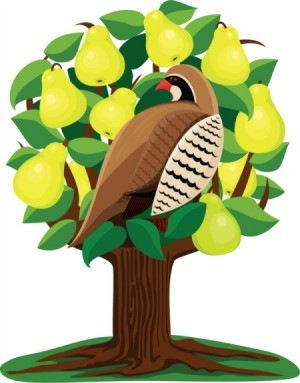# Day 1: A Partridge in a Number TreeA partridge sits in its tree. However, this is not a pear tree; it is a number tree! It rummages around until it finds a special type of number- let us call them partridge numbers.

Define a partridge number to be a number such that:

1. It is a square number,
2. It can be expressed in the form $x^2y^2-x^2-y^2+2$ where the positive integers $x$ and $y$ are not consecutive,
3. It cannot be expressed in the form $x^2y^2-x^2-y^2+2$ for any consecutive positive integers $x$ and $y$.

The first partridge number is between $1$ and $2014$. What number is it?

This problem is part of the set Advent Calendar 2014.

×